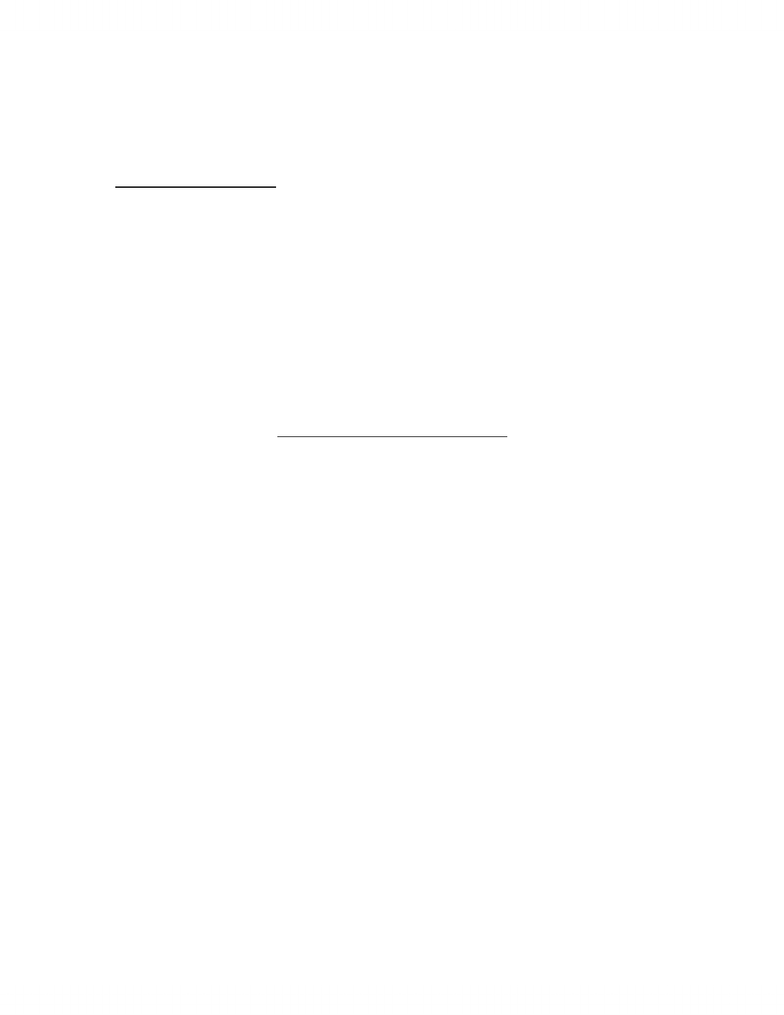Textbook Notes (280,000)
CA (160,000)
UW (6,000)
ECON (500)
ECON101 (200)
Chapter 4

ECON101 Chapter Notes - Chapter 4: Demand Curve, Economic Equilibrium, Negative Number

Department
Economics
Course Code
ECON101
Professor
Shi Lei Niu
Chapter
4

This preview shows pages 1-2. to view the full 6 pages of the document.ECON 101 Notes
Chapter 4 Elasticity
Price Elasticity of Demand
- When supply increases, the equilibrium price falls and the equilibrium quantity
increases
- Price changes depends on responsiveness of quantity demanded to a change in price
- Responsiveness depends on slope
- Slope of a demand curve depends on the units in which we measure the price and the
quantity
- The price elasticity of demand is a units-free measure of the responsiveness of the
quantity demanded if a good to a change in its price when all other influences on
buyers’ plans remain the same
Calculating Price Elasticity of Demand
Price elasticity of demand = percentage change in quantity demanded
percentage change in price
- To use this formula, we need to know the quantities demanded at different prices when
all other incluences on buyers’ plans remain the same
- To calculate the price elasticity of demand, we express the changes in price and quantity
demanded as percentages of the average price and the average quantity
- Average price and quantity gives most precise measurement of elasticity at the
midpoint between original price and new price
Percentages and Proportions
- Elasticity is the ratio of two percentage changes
- A percentage change is a proportionate change multiplied by 100
- The proportionate change in price is change in price / average price, and the
proportionate change in quantity is change in quantity / average quantity
A Units-Free Measure
- Elasticity is a units-free measure because the percentage change in each variable is
independent of the units in which the variable is measured
- The ratio of two percentages is a number without units
Minus Sign and Elasticity
- When the price of a good rises, the quantity demanded decreases along the demand
curve
- Because a positive change in price brings a negative change in the quantity demanded,
the price elasticity of demand is a negative number
- It is the magnitude, or absolute value, of the price elasticity of demand that tell us how
responsive, how elastic, demand is

Only pages 1-2 are available for preview. Some parts have been intentionally blurred.- To compare the price elasticities of demand, we us the magnitude of the elasticity and
ignore the minus sign
Inelastic and Elastic Demand
- If the quantity demanded remains constant when the price changes, then the price
elasticity of demand is zero and the good is said to have a perfectly inelastic demand
- If the percentage change in the quantity demanded equals the percentage change in
price, then the price elasticity equals 1 and the good is said to have a unit elastic
demand
- If the elasticity of demand is between zero and 1 then the good is said to have an
inelastic demand
- If the quantity demanded changes by an infinitely large percentage in response to a tiny
price change, then the price elasticity of demand is infinity and the good is said to have
a perfectly elastic demand
- If the price elasticity of demand is greater than 1 then the good is said to have an elastic
demand
Elasticity Along a Straight-Line Demand Curve
- Elasticity and slope are not the same, but they are related
- At the midpoint of the curve, demand is unit elastic
- Above midpoint, demand is elastic
- Below midpoint, demand is inelastic
Total Revenue and Elasticity
- The total revenue from sale of a good equals the price of the good multiplied by the
quantity sold
- When a price changes, total revenue also changes
- A rise in price does not always increase total revenue
Change in total revenue depends on elasticity of demand:
- If demand is elastic, 1 percent price cut increases the quantity sold by more than 1
percent and total revenue increases
- If demand is inelastic, 1 percent price cut increases the quantity sold by less than 1
percent and total revenue decreases
- If demand is unit elastic, 1 percent price cut increases the quantity sold by 1 percent and
so total revenue does not change
- The total revenue test is a method of estimating the price elasticity of demand by
observing the change in total revenue that results from a change in price, when all other
influences on the quantity sold remain the same
- If a price cut increases total revenue, demand is elastic
- If a price cut decreases total revenue, demand is inelastic
- If a price cut leaves total revenue unchanged, demand is unit elastic
You're Reading a Preview

Unlock to view full version